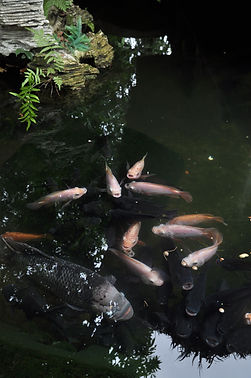### Koi Pond Stocking Guide

There is no definitive answer to the question, "How many Koi can I keep in my pond?"

This is because of the fact every pond is different particularly when it comes to surface area, filtration systems and pump capacities.

As a rule of thumb if you work on the basis of keeping 55cm of Koi per 1000 litres of pond water, and your filtration is of adequate size then this will be a good starting point.

For example if you had a pond volume of 5000 litres you could keep a maximum of 275 cm of fish, bearing in mind that the fish you buy from your shop are likely to be juveniles and therefore likely to grow substantially more. For a useful guide to your pond click on the link below:

http://worldofwater.com/how-to-ponds/#### How much water is in my pond?

To work out the volume of the pond water in your pond there are two calculations, which depend on if you work in metric or imperial.

The easiest of the two is metric as one cubic metre is equal to 1000 litres.

So for example if your pond measured 3 metres in length by 2 metres wide and 1 metre deep you would multiply 3x2x1=6 (6000 litres) so the amount in your pond is Length x Width x Depth = Cubic Metres in your pond.

If you prefer to work in gallons however you need to measure the maximum width, length and depth in feet using the following formula.

So for example if your pond measured 12 foot in length by 8 foot wide and a depth of 3 foot you would multiply 12x8x3 (but also multiply by 6.25) therefore 12x8x3 = 288x6.25 = 1800 gallons in your pond.